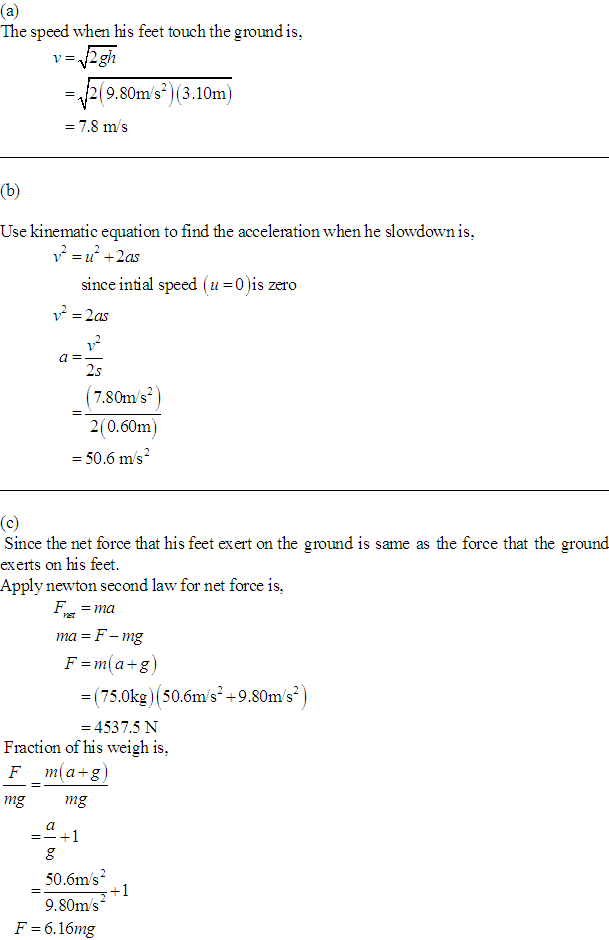A 75.0-kg man steps off a platform 3.10 m above the ground. Hekeeps his legs straight as he falls, but at the moment his feettouch the ground his kneeBrittney Lord 2021-03-12 Answered
A 75.0-kg man steps off a platform 3.10 m above the ground. Hekeeps his legs straight as he falls, but at the moment his feettouch the ground his knees begin to bend, and, treated as aparticle, he moves an additional 0.60 m before coming torest.
a) what is the speed at the instant his feet touch theground?
b) treating him as a particle, what is his acceleration(magnitude and direction) as he slows down, if the acceleration isassumed to be constant?
c) draw his free-body diagram (see section 4.6). in termsof forces on the diagram, what is the net force on him? usenewton's laws and the results of part (b) to calculate the averageforce his feet exert on the ground while he slows down. expressthis force in newtons and also as a multiple of his weight.

• Questions are typically answered in as fast as 30 minutes

Solve your problem for the price of one coffee

• Math expert for every subject
• Pay only if we can solve itLeonard Stokes
Here are the detailed explanations:Not exactly what you’re looking for?content_user

a) The speed when his feet touch the ground is,

$$v=\sqrt{2gh}$$

$$=\sqrt{2(9.80\ m/s^2)(3.10m)}$$

$$=7.8\ m/s$$

b) Use kinematic equation to find the acceleration when he showdown is,

$$v^2=u^2+2as$$

since initial speed (u=0) is zero

$$v^2=2as$$

$$a=\frac{v^2}{2s}$$

$$=\frac{(7.80\ m/s^2)}{2(0.60\ m)}$$

$$=50.6\ m/s^2$$

c) Since the net force that his feet exert on the ground is same as the force that the ground exerts on his feet.

Apply newton second law for net force is,

$$F_{m}=ma$$

$$ma=F-mg$$

$$F=m(a+g)$$

$$=75.0\ kg)(50.6\ m/s^2+9.80\ m/s^2)$$

$$=4537.5\ N$$

Fraction of his weigh is,

$$\frac{F}{mg}=\frac{m(a+g)}{mg}$$

$$=\frac{a}{g}+1$$

$$=\frac{50.6\ m/s^2}{9.80\ m/s^2}+1$$

$$F=6.16mg$$